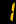# Primary Curriculum

## Primary 1

• Numbers to 10
• Numbers to 20
• 3D Shapes (I)
• Straight lines and curves
• Length and distance (I)
• Numbers to 100
• 2D Shapes
• Hong Kong money (I)
• Length and distance (II)
• Time (I)

## Primary 2

• 3-digit numbers
• Basic Multiplication
• 3D shapes (II)
• Angles (I)
• Length and distance (III)
• Time (II)
• 4-digit numbers
• Basic Division
• The four directions
• Hong Kong money (II)
• Weight
• Pictorgrams (I)

## Primary 3

• 5-digit numbers
• Multiplication (I)
• Division (I)
• Parallel and Perpendicular
• Length and distance (IV)
• Time (III)
• Mixed operations (I)
• Fractions (I)
• Angles (II)
• Triangles
• Capacity
• Time (IV)
• Block graphs

## Primary 4

• Multiplication (II)
• Division (II)
• Acquaintance with modern calculation devices
• Multiples and factors
• Common multiples and common factors
• Perimeter (I)
• Mixed operations (II)
• Fractions (II)
• Decimals (I)
• Fitting and dissecting shapes
• Symmetry
• Area(I)
• Bar charts (I)

## Primary 5

• Large numbers
• Fractions (III)
• Fractions (IV)
• The eight compass points
• Area (II)
• Pictograms (II)
• Elementary algebra
• Decimals (II)
• Decimals (III)
• Fractions (V)
• 3D shapes (III)
• Volume (I)
• Bar charts (II)
• Simple equations (I)

## Primary 6

• Decimals (IV)
• Decimals (V)
• Percentages (I)
• 3D Shape (IV)
• Volume (II)
• Averages
• Bar charts (III)
• Percentages (II)
• Circles
• Perimeter (II)
• Speed
• Broken line graphs
• Simple equations (II)